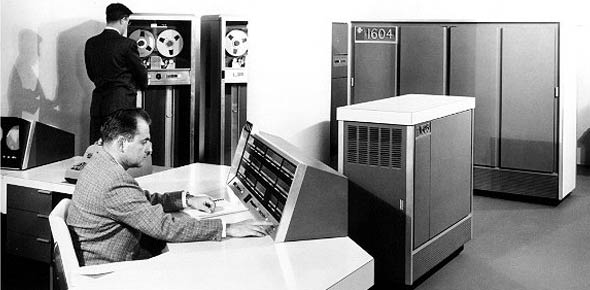# CDC 2EX5X Communications-electronics Journeyman Part 2

140 Questions | Total Attempts: 526SettingsContiuation of 2EX5X Pretest Part 1.

• 1.
What principle does the time domain reflectometer use to test cables?
• A.

• 2.
What secondary cell is prone to the "memory effect"?
• A.

• 3.
What part of the power generation process allows a smooth transition of electrical current between multiple sources and the load?
• A.

• 4.
What deployable communication system is a state of the art ground-to-ground communications infrastructure designed to exchanged secure and non-secure voice, data, and video communications via line of sight, satellite, or hard wired resources?
• A.

• 5.
What are the two sampling techniques of a digital storage oscilloscope?
• A.

Real-time and repetitive sampling

• B.

Real-time and averaging sampling

• C.

Repetitive and averaging sampling

• D.

Linear and averaging sampling

• 6.
What are the two types of intepolation?
• A.

Real-time and linear

• B.

Real-time and sine

• C.

Sine and Linear

• D.

Sine and Repetitive

• 7.
Which is not a type of acquisition mode on the digital storage oscilloscope?
• A.

Sample Mode

• B.

Repetitive Mode

• C.

Peak Detection Mode

• D.

Averaging Mode

• 8.
What is teh frequency range of an audio signal generator?
• A.

10Hz to 10kHz

• B.

20Hz to 20kHz

• C.

10kHz to 10GHz

• D.

20kHz to 35MHz

• 9.
Which one of these is not an application of a radio frequency generator?
• A.

Verifying transmitter frequencies

• B.

• C.

Checking antenna systems

• D.

Aligning galvanometers

• 10.
A radio frequency generator is used to
• A.

Monitor odd harmonics

• B.

• C.

Modulate subcarrier oscillators

• D.

Check galvanometer frequency response

• 11.
What is the variable voltage range of signals output from the HP 3325B synthesizer/function generator?
• A.

1 mV to 10 V peak-to-peak

• B.

1 mV to 10V peak

• C.

1 V to 10V peak-to-peak

• D.

1V to 10V peak

• 12.
The output of the HP 3325B function generator may generate only DC voltages, to a maximum of
• A.

+/- 1V

• B.

+/- 4.5V

• C.

+/- 5V

• D.

+/- 10V

• 13.
In using the phase modulation controls of the HP3325B function generator, an input of a +/- 5 V peak would result in what approximate phase deviation?
• A.

+/- 42.5 degrees per volt for sine wave

• B.

+/- 42.5 degrees per volt for square wave

• C.

+/- 425 degrees per volt for sine wave

• D.

+/- 425 degrees per volt for square wave

• 14.
The 10:1 power ratio represented by 1 bel can also be represented by how many decibels (db)?
• A.

1 dB

• B.

3dB

• C.

6 dB

• D.

10 dB

• 15.
A power reading that uses the abbreviation dBm indicates the
• A.

Ratio of decibels relative to a 1-milliwatt standard

• B.

Ratio of decibels relative to a 1-megawatt standard

• C.

Actual power level expressed in milliwatts

• D.

Actual power level expressed in megawatts

• 16.
The measurable frequency and power ranges of the HP 436A power meter are determined by the
• A.

Digital display

• B.

Power sensor

• C.

Power meter

• D.

Interface

• 17.
During what operation is the radio frequency blanking output used on the HP 436A power meter?
• A.

While in WATT mode operation

• B.

While in REMOTE mode operation

• C.

During the automatice zeroing operation

• D.

• 18.
Which measurements is not made with a spectrum analyzer?
• A.

Frequency stability

• B.

Peak-peak voltage

• C.

Amplitude modulation

• D.

Subcarrier oscillator output

• 19.
Which measurements do you make with a spectrum analyzer?
• A.

Subcarrier oscillator outputs

• B.

Transducer output voltage

• C.

Time between two events

• D.

Pulse width

• 20.
Which electronic counter measurement do you make with a spectrum analyzer?
• A.

Period

• B.

Scaling

• C.

Frequency

• D.

Time interval

• 21.
Which electronic counter measurement counts the number of bits in a pulse train?
• A.

Period

• B.

Scaling

• C.

Totalize

• D.

Time interval

• 22.
Which are the correct four classes of electronic counters?
• A.

Frequency counters, time counters, microwave counters, and reciprocal counters

• B.

Frequency counters, universal counters, microwave counters, and reciprocal counters

• C.

Frequency counters, universal counters, period counters, and reciprocal counters

• D.

Scaling counters, universal counters, microwave counters, and reciprocal counters

• 23.
Which push-button(s)  would you select to get 125 ohm impedance on the time domain reflectometer?
• A.

None

• B.

75 ohm only

• C.

125 ohm only

• D.

50 ohm and 75 ohm

• 24.
What tye of deflection return on the TDR CRT would indicate a cable "short?'
• A.

No "pip"

• B.

Smear "pip"

• C.

Upward deflections "pip"

• D.

Downward deflections "pip"

• 25.
While using a time domain reflectometer (TDR), what cable fault causes delays in pulses of propagation velocity making accurate measurements difficult?
• A.

Short

• B.

Open

• C.

Grounded

• D.

Moisture in cables

Related TopicsBack to top